# 前言

``async function recursive() {``  if( active ) return;``  // do something``  await recursive();``}``

# 发现问题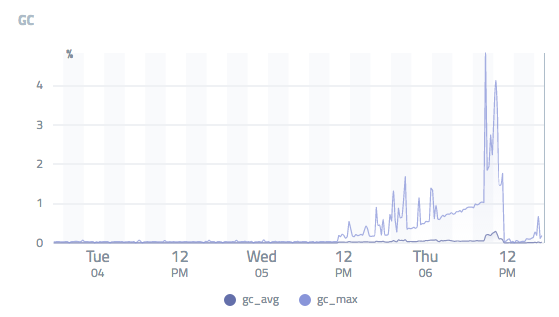# 定位问题

## 堆快照分析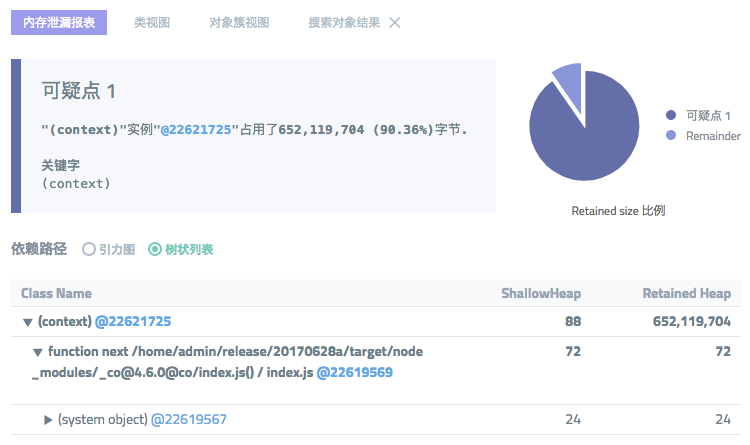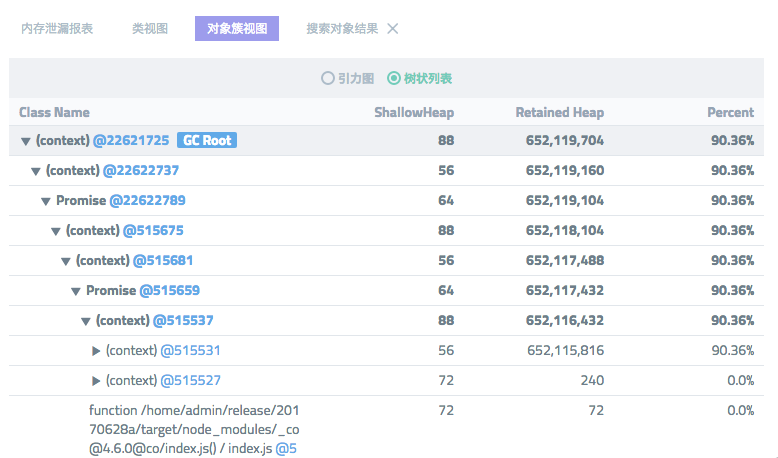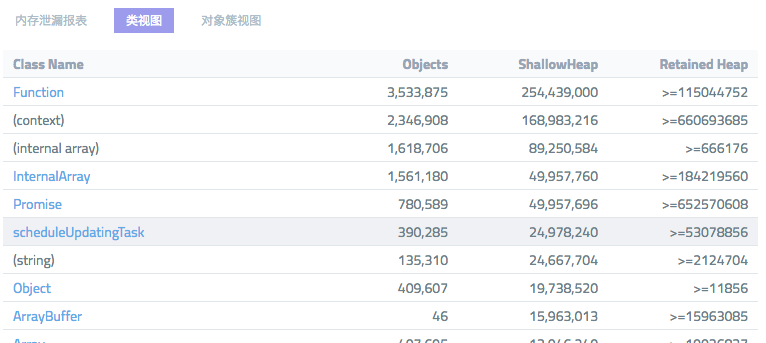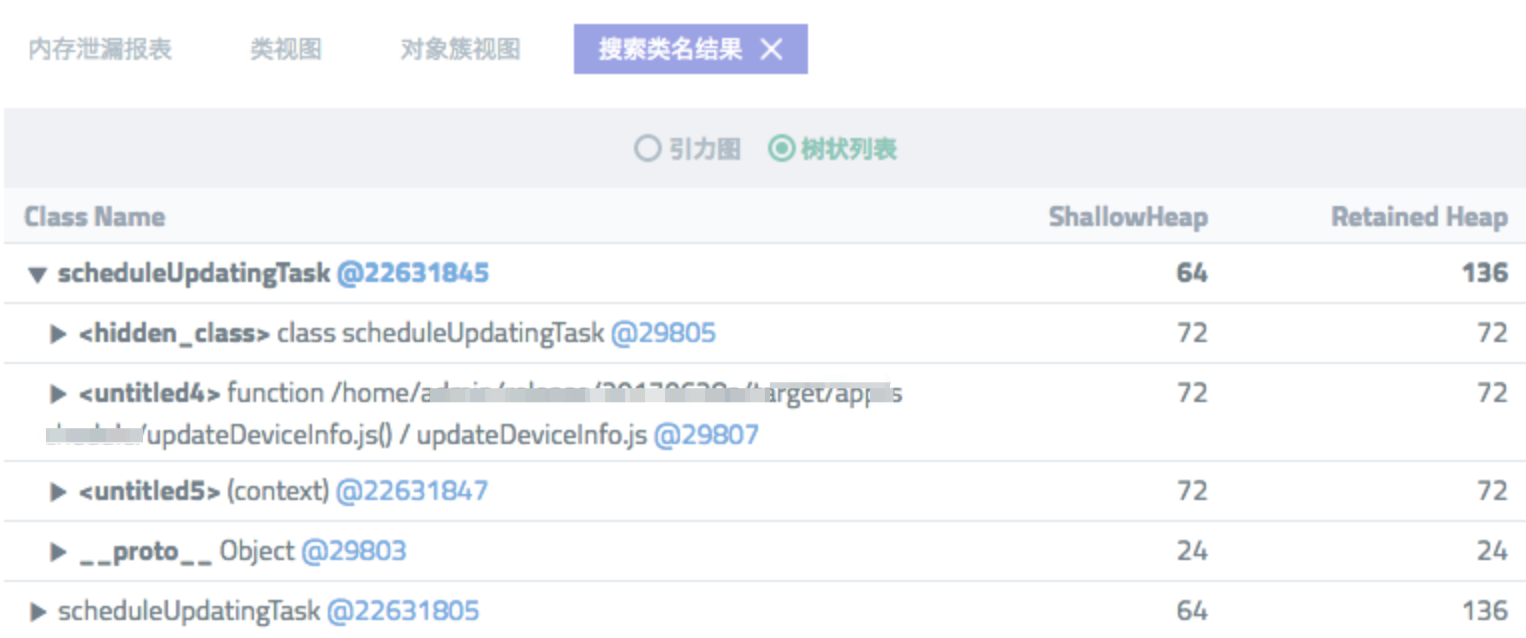# 代码分析

``// 执行业务，成功之后稍作等待，继续``// 如果拿锁失败了，停止``const scheduleUpdatingTask = function* (ctx) {``  if (!taskActive) return;``  try {``    yield doSomething(ctx);``  } catch (e) {``    // 需要捕获业务异常，即使挂了，下一次schedule也能正常跑``    ctx.logger.error(e);``  }``  yield scheduleUpdatingTask(ctx);``};``

``const co = require('co');``function sleep(ms) {``  return new Promise((resolve) => {``    setTimeout(() => {``      resolve();``    }, ms);``  });``}``function* task() {``  yield sleep(2);``  console.log(process.memoryUsage());``  yield task();``}``co(function* () {``  yield task();``});``

``function sleep(ms) {``  return new Promise((resolve) => {``    setTimeout(() => {``      resolve();``    }, ms);``  });``}``async function task() {``  await sleep(2);``  console.log(process.memoryUsage());``  await task();``}``task();``

# 解决问题

``async function task() {``  while (true) {``    await sleep(2);``    console.log(process.memoryUsage());``  }``}``